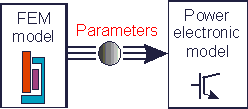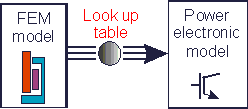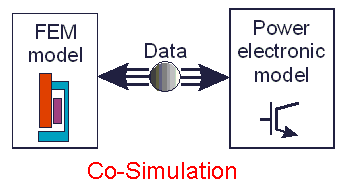• Introduction
• What is in this manual
• What is Caspoc
• User interface
• Introduction
• Starting
• Simulation
• Editing
• Viewing and printing
• Getting Started
• Basic editing
• Simulation in the time domain
• Basic User Interface Topics
• Editing
• Simulation
• Viewing
• Library
• Reports
• Project management
• Circuit and Block Diagram Components
• Introduction
• Cscript and user defined functions
• Component parameters
• Modeling Topics
• Introduction
• Power Electronics
• Semiconductors
• Electrical Machines
• Electrical drives
• Power Systems
• Mechanical Systems
• Thermal Systems
• Magnetic Circuits
• Green Energy
• Coupling to FEM
• Experimenter
• Analog hardware description language
• Embedded C code Export
• Coupling to Spice
• Small Signal Analysis
• Matlab coupling
• Tips and tricks
• Appendices

## FEM Coupling Introduction.

Electrical machines are non-linear components that form the interface from the power electronics with control to the mechanical system. Electrical machines are optimized for the electromagnetic design and to some extend for their thermal behavior, but their non-linear behavior due to, for example, cogging torque or torque ripple is not included in the overall system analysis.

Linear models for integration into system analysis can be used to model electrical machines. Even for these simple machine models there is still the question where we get the parameters. Also non-linear effects like saturation or tooth harmonics are not included.

Using simple linear models can already help to study the behavior of electrical drives. For example, in a Switched Reluctance Machine (SRM) simulation the rotor angular position dependent torque, inductance and back emf is required. Using Finite Element software the non-linear effects in electrical machines can be analysed, but still need to be incorporated into the entire model. There are basically three methods.

1. Static parameters for inductance, torque constant and back emf, are measured from static FEM analysis.2. Flux linkage or co-energy tables from static FEM analysis can be created that are used in the system simulation using look up tables.3. Co-simulation between FEM and the system simulation.Only by using the co-simulation all non-linear effects, like tooth-tip saturation of stator and rotor poles and eddy currents can be taken into account.